Next: Soft SUSY Breaking Terms Up: Model Independent Results Previous: Model Independent Results

## Gaugino Condensation

The idea of breaking supersymmetry in a dynamical way was first presented in refs. . In those articles a general topological argument was developed in terms of the Witten index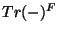, showing that dynamical supersymmetry breaking cannot be achieved unless there is chiral matter or we include supergravity effects for which the index argument does not apply. This was subsequently verified by explicitly studying gaugino condensation in pure supersymmetric Yang-Mills, a vector-like theory, for which gauginos condense but do not break global supersymmetry  (for a review see ). Breaking global supersymmetry with chiral matter was an open possibility in principle, but this approach ran into many problems when tried to be realized in practice.

The situation improved very much with the coupling to supergravity. The reason was that simple gaugino condensation was argued to be sufficient to break supersymmetry once the coupling to gravity was included. This works in a hidden sector mechanism where gravity is the messenger of supersymmetry breaking to the observable sector . However, it has recently been realized that the proposal in  does not work (see for instance  which also includes an extended discussion of the current status of gaugino condensation); coupling with supergravity does not actually change the situation in global supersymmetry where gaugino condensation does not break supersymmetry. The missing ingredient was the fact that gauge couplings were considered to be constant rather than field dependent. Gaugino condensation with field dependent gauge couplings was anticipated in ref.  and is realized in a very natural way in string theory. As we have seen, the gauge coupling is a function of the dilaton and moduli fields. Furthermore, string theory provides a natural realization of the hidden sector models [77,78] by having a hidden sector especially in the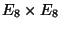versions.

To study the effects of gaugino condensation we should be able to answer the following questions: Do gauginos condense? If so, is supersymmetry broken by this effect? What is the effective theory below the scale of condensation? In order to answer these questions, several ideas have been put forward [72,76,78,79,80,81]. The most convenient formalism is in terms of the so-called 2PI effective action . In order to understand this formalism, it is convenient to think about the case of spontaneous breaking of gauge symmetries. In that case we minimize the effective potential for a Higgs field, obtained from the 1PI effective action and see if the minimum breaks or not the corresponding gauge symmetry. In our case, we are interested in the expectation value of a composite field, namely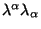or its supersymmetric expression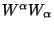. Therefore we need the two particle irreducible effective action (2PI).

We start then with the generating functional in the presence of an external current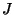coupled to the operator that we want the expectation value of, namely,. Let us consider the simplest case of a single hidden sector gauge group in global supersymmetry. (Coupling to supergravity presents no obstacles but make the discussion more cumbersome [81,75].)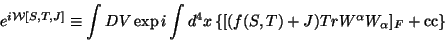(37)

From this we have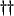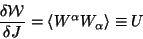(38)

and define the 2PI action as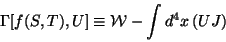(39)

To find the explicit form of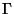we use the fact that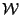depends on its two arguments only through the combination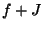, therefore, we can see that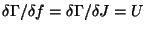. Integrating this equation implies thathas to be linear in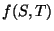: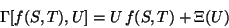(40)

where the arbitrary function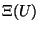can be determined using symmetry arguments as in [72,49,81]. We find: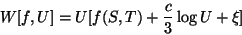(41)

Here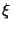is an arbitrary constant. We can see that the superpotential corresponds to the one found in . Therefore this 2PI action is a reinterpretation of the one in . We have to stress that in our treatment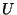is only a classical field, not to be integrated out in any path integral. It also does not make sense to consider loop corrections to its potential, this solves the question raised in  where loop corrections to thepotential could change the tree level results. Furthermore, sinceis classical we can eliminate it by just solving its field equations: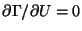. These equations cannot be solved explicitly but we find the solution in an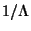expansion, with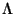the scale of condensation . We find that the solution of these equations reproduces at first order, the Wilson action derived in , for which we can just read the superpotential (using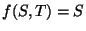) to be: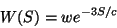(42)

where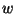is an arbitrary constant. The superpotential is just the one found in . The correction to the Kähler potential is not completely known due mainly to the fact that the perturbative corrections to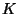are completely unknown . Notice that these are corrections of order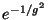as expected.

By studying the effective potential forwe recover the previously known results. For one condensate and field independent gauge couplings (no field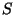) the gauginos condense (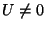) but supersymmetry is unbroken. For field dependendt gauge coupling, the minimum is for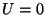(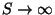) so gauginos do not condense (this is reflected in the runaway behaviour of the Wilsonian action for).

Alternatively, after eliminatingfrom its field equations and using (42), we find the scalar potential for the real parts ofand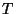(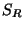and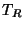respectively), namely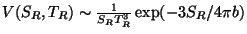. This potential has a runaway behaviour for bothand, as expected.

Thedependence of the potential was completely changed after the consideration of target space orduality. It was shown , that imposing this symmetry changes the structure of the scalar potential for the moduli fields in such a way that it develops a minimum at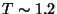(in string units), whereas the potential blows-up at the decompactification limit (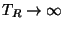), as desired (see figure 3)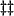. The modifications due to imposingduality can be traced to the fact that the gauge couplings get moduli dependent threshold corrections from loops of heavy string states  as in eq. (29). This in turn generates a moduli dependence on the superpotential induced by gaugino condensation of the form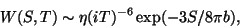(43)

with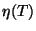the Dedekind function. This formula is actually more complicated if the coefficients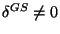in which casealso transform under T-duality as in eq. (34), see for instance B. de Carlos et al in .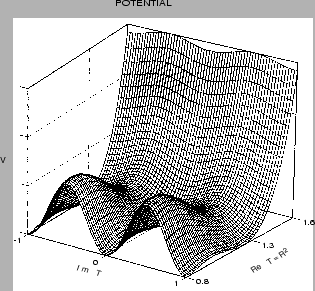This mechanism however did not help in changing the runaway behaviour of the potential in the direction of. There is a very generic problem emphasized mostly by Dine and Seiberg  . It is known that because at largethe string is weakly coupled, the potential has to vanish asymptotically (towards a free theory). Any other minimum has to be at strong coupling for which the perturbation expansion does not work, unless there is an extra parameter that could be tuned. Such a mechanism was proposed in . For stabilizing, the proposal was to consider gaugino condensation of a nonsemisimple gauge group, inducing a sum of exponentials in the superpotential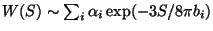which can conspire to generate a local minimum for. The role of the extra parameter can be played by the ratio of beta function coefficients of the different groups. These have been named racetrack' models in the recent literature.

It was later found that combining the previous ideas, together with the addition of matter fields in the hidden sector (natural in many string models)[86,87], was sufficient to find a minimum with almost all the right properties, namely,andfixed at the desired value,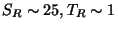, supersymmetry broken at a small scale (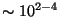GeV) in the observable sector, etc. This lead to studies of the induced soft breaking terms at low energies. Besides that relative success there are several problems that assure us that we are far from a satisfactory description of these issues.

Another important puzzle was: we know that the fieldonly appears after performing a duality transformation changing the stringy field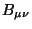to the axion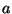. A non-trivial potential forgives a mass toand then it is no longer dual to! This puzzle was recently solved  by analyzing gaugino condensation directly in theversion. The end result was thatdissapears from the low-energy spectrum and a massive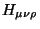field takes its place, having one propagating degree of freedom and being dual to a massive axion.

(i)
Unlike the case for, fixing the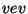of the dilaton field, at the phenomenologically interesting value, is not achieved in a satisfactory way. The conspiracy of several condensates with hidden matter to generate a local minimum at a good value, requires certain amount of fine tunning and cannot be called natural. One of the motivations for proposing the existence of a-duality was precisely to find a way in which the vev ofcould be fixed in a natural way as it happens for. More recently, there have been attempts to combine gaugino condensation withduality , in whichcan be fixed at a selfdual point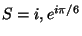; but, besides the present ignorance of how-duality can be realized in 4D effective actions, there is also an unjustified assumption that this effect will provide the dominant non-perturbative correction to the superpotential (see for instance  for a discussion of these points). Possible arguments for this to be the case were given in . There, it was also proposed to use the recently found non-perturbative behaviour of string models, of the form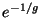(rather than the field theoretical). These corrections in the Kähler potential can in principle combine with a superpotential like that of eqs. (42,43), to fix the value of. There is no yet a concrete case where these ideas are realized, though.

(ii)
The cosmological constant turns out to be always negative, which looks like an unsourmountable problem at present. This also makes the analysis of soft breaking terms less reliable, because in order to talk about them, a constant piece has to be added to the Lagrangian that cancels the cosmological constant. It is then hard to believe that the unknown mechanism generating this term would leave the results on soft breaking terms (such as the smallness of gaugino masses) untouched.

(iii)
Finally, even if the previous problems were solved, there are at least two serious cosmological problems for the gaugino condensation scenario. First, it was found under very general grounds, that it was not possible to get inflation with the type of dilaton potentials obtained from gaugino condensation . Second is the so-called cosmological moduli problem' which applies to any (non-renormalizble) hidden sector scenario including gaugino condensation . In this case, it can be shown that if the same effect that fixes the vev's of the moduli, also breaks supersymmetry, then: the moduli and dilaton fields acquire masses of the electroweak scale (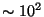GeV) after supersymmetry breaking . Therefore if stable, they overclose the universe, if unstable, they destroy nucleosynthesis by their late decay, since they only have gravitational strength interactions. At present there is no satisfactory explanation of this problem and it stands as one of the unsolved generic problems of string phenomenology.Next: Soft SUSY Breaking Terms Up: Model Independent Results Previous: Model Independent Results
root 2001-01-22